# numpy.linalg.cholesky¶

numpy.linalg.cholesky(a)[source]

Cholesky decomposition.

Return the Cholesky decomposition, L * L.H, of the square matrix a, where L is lower-triangular and .H is the conjugate transpose operator (which is the ordinary transpose if a is real-valued). a must be Hermitian (symmetric if real-valued) and positive-definite. Only L is actually returned.

Parameters: a : (..., M, M) array_like Hermitian (symmetric if all elements are real), positive-definite input matrix. L : (..., M, M) array_like Upper or lower-triangular Cholesky factor of a. Returns a matrix object if a is a matrix object. LinAlgError If the decomposition fails, for example, if a is not positive-definite.

Notes

New in version 1.8.0.

Broadcasting rules apply, see the numpy.linalg documentation for details.

The Cholesky decomposition is often used as a fast way of solving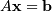(when A is both Hermitian/symmetric and positive-definite).

First, we solve for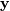in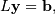and then for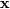in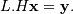Examples

>>> A = np.array([[1,-2j],[2j,5]])
>>> A
array([[ 1.+0.j,  0.-2.j],
[ 0.+2.j,  5.+0.j]])
>>> L = np.linalg.cholesky(A)
>>> L
array([[ 1.+0.j,  0.+0.j],
[ 0.+2.j,  1.+0.j]])
>>> np.dot(L, L.T.conj()) # verify that L * L.H = A
array([[ 1.+0.j,  0.-2.j],
[ 0.+2.j,  5.+0.j]])
>>> A = [[1,-2j],[2j,5]] # what happens if A is only array_like?
>>> np.linalg.cholesky(A) # an ndarray object is returned
array([[ 1.+0.j,  0.+0.j],
[ 0.+2.j,  1.+0.j]])
>>> # But a matrix object is returned if A is a matrix object
>>> LA.cholesky(np.matrix(A))
matrix([[ 1.+0.j,  0.+0.j],
[ 0.+2.j,  1.+0.j]])


numpy.kron

numpy.linalg.qr